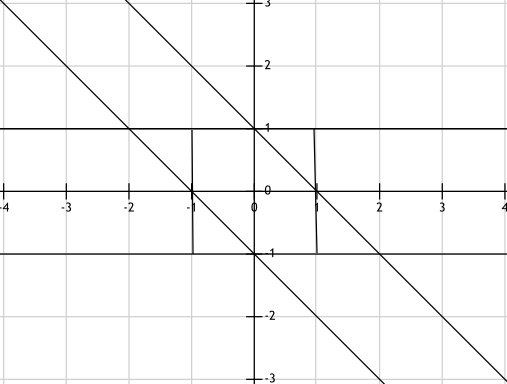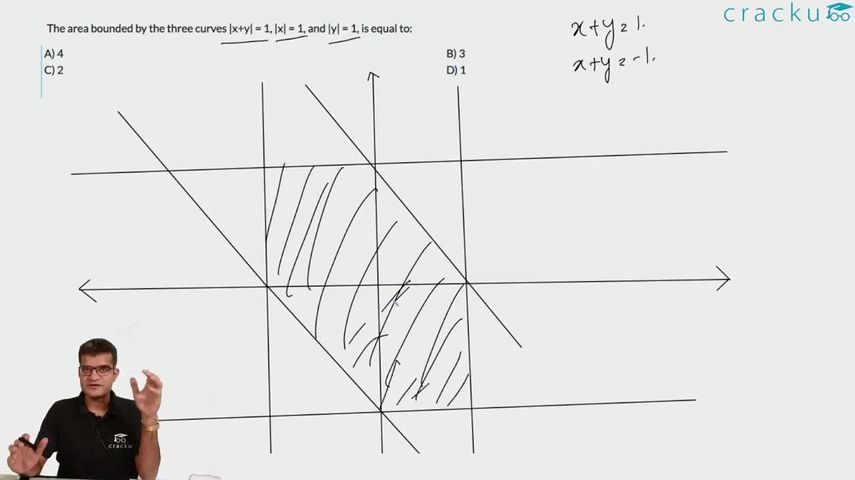Question 149

# The area bounded by the three curves |x+y| = 1, |x| = 1, and |y| = 1, is equal to:

Solution

|x| = 1 and |y| = 1 form a square of area = 2*2 = 4 sq units

|x+y| = 1 forms a set of parallel lines cutting the axes at (1,0), (0,1), (-1,0) and (0,-1). The graph is as shown:The area bounded by the three curves is 2*2 - 1/2*1*1*2 = 4 - 1 = 3 sq units

### View Video Solution